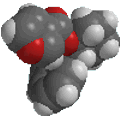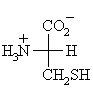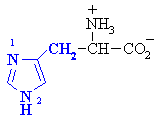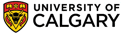Chapter 27: Amino Acids, Peptides and ProteinsAmino Acids questions

 Qu 1: Why are the non-polar side chains unreactive ? Qu 2: Does L-cysteine have R- or S- absolute configuration ?L-cysteine Qu 3: Regular carboxylic acids such as ethanoic acid, have pKas around 5, while in α-amino acids, the carboxyl pKa =2.  Why is this ? Qu 4: The amino acid histidine has two potentially basic N sites in the side chain, which one is more basic and why ?Qu 5: What is the isoelectronic point, pI, of each of the following hypothetical amino acids for which you are given their pKa values ? (pKa1 = carboxyl,  pKa2 = ammonium, pKa3 = side chain functional group) (a) pKa1 = 2.0,  pKa2 = 10.0  (b) pKa1 = 2.0,  pKa2 = 10.0,  pKa3 = 4.0  (c) pKa1 = 2.0,  pKa2 = 10.0,  pKa3 = 8.0© Dr. Ian Hunt, Department of Chemistry